# RS Aggarwal Solutions for Class 9 Chapter 15: Volume and Surface Area of Solids Exercise 15A

## RS Aggarwal Solutions for Class 9 Maths Exercise 15A PDF

RS Aggarwal Solutions for Class 9 contains accurate solutions for the exercise wise problems based on the CBSE syllabus. The solutions are prepared in such a way they help students to perform better in the board exams. The students can make use of solutions as a reference material to get their doubts cleared in an efficient manner. The solutions are prepared by experienced faculty having vast teaching experience in Mathematics to serve the purpose. RS Aggarwal Solutions for Class 9 Maths Chapter 15 Volume and Surface Area of Solids Exercise 15A are provided here.

## RS Aggarwal Solutions for Class 9 Chapter 15: Volume and Surface Area of Solids Exercise 15A Download PDF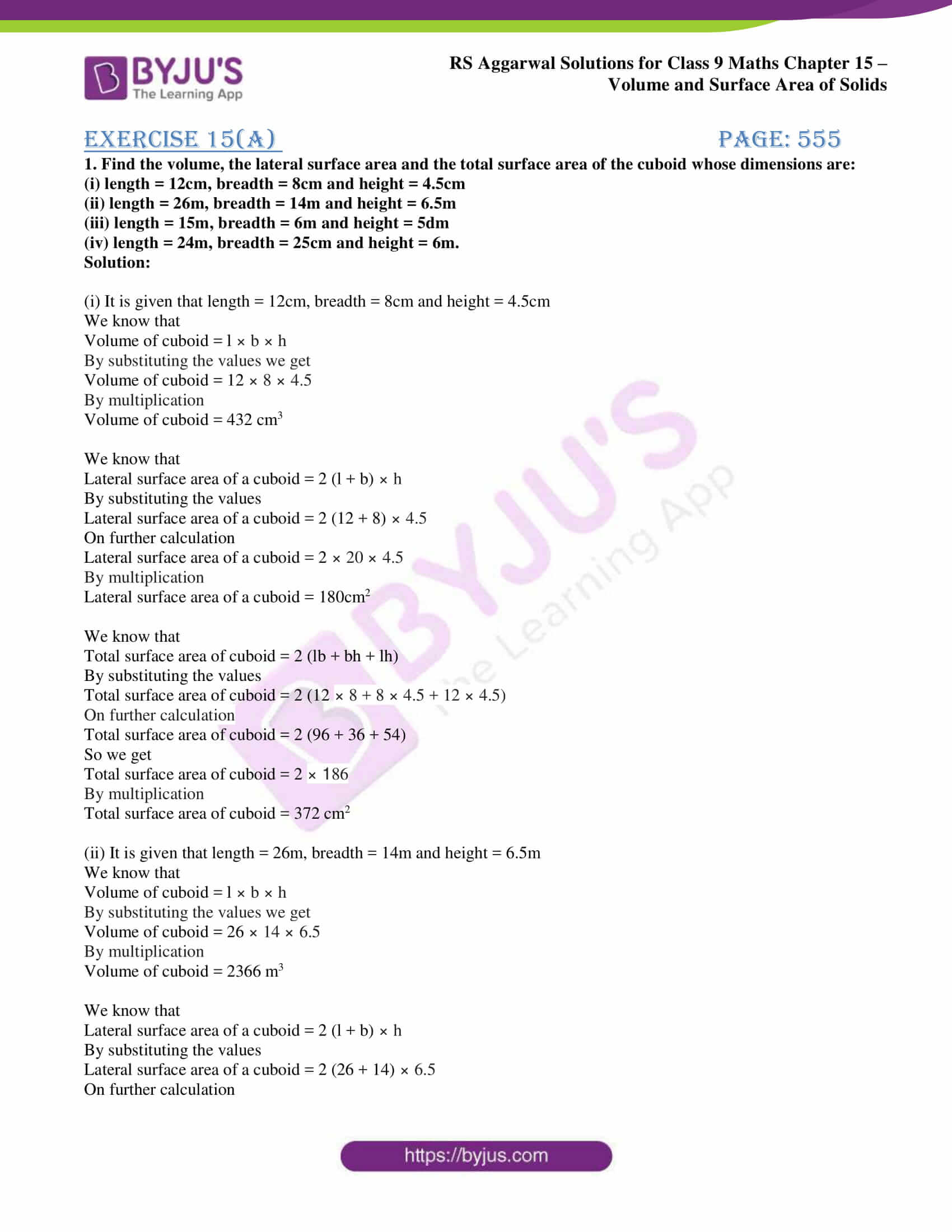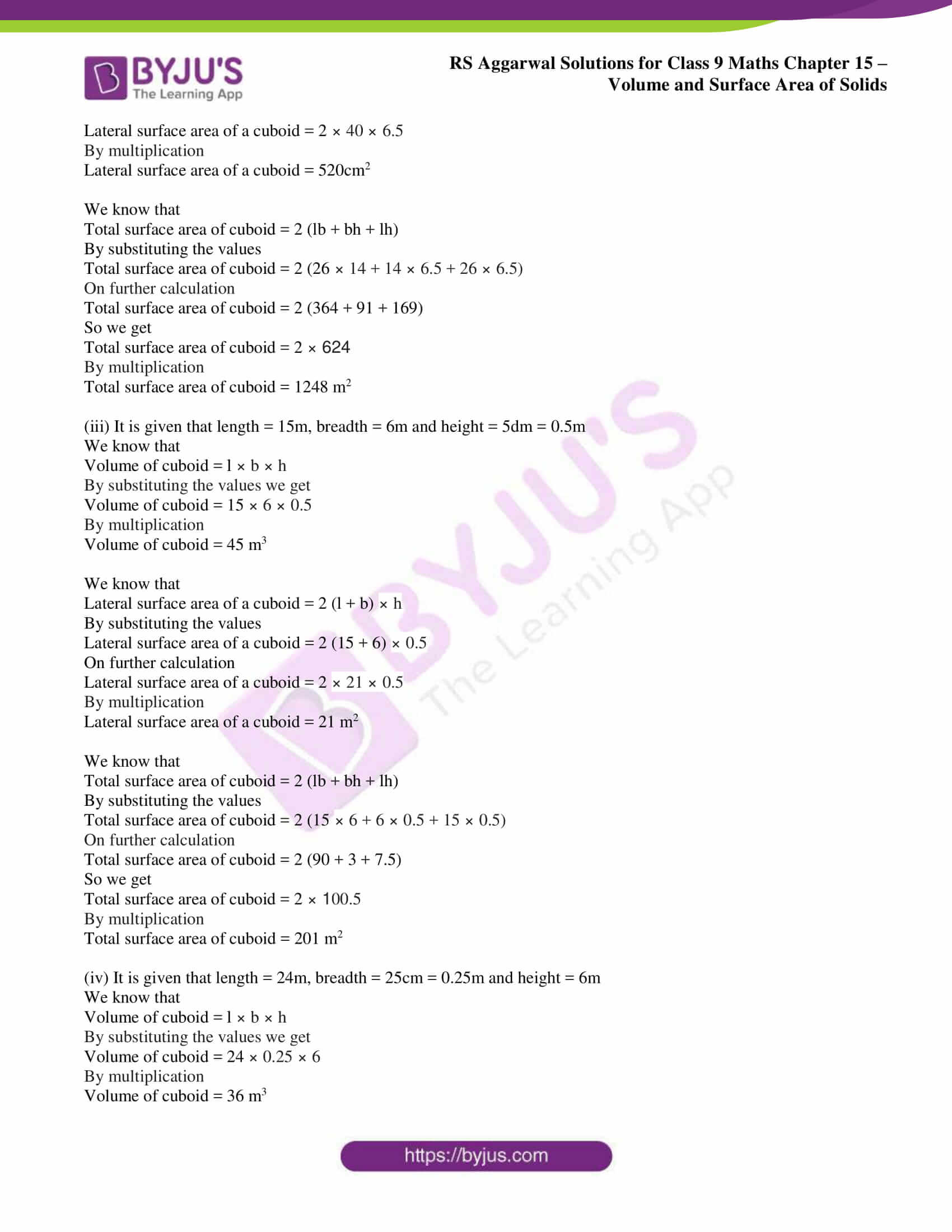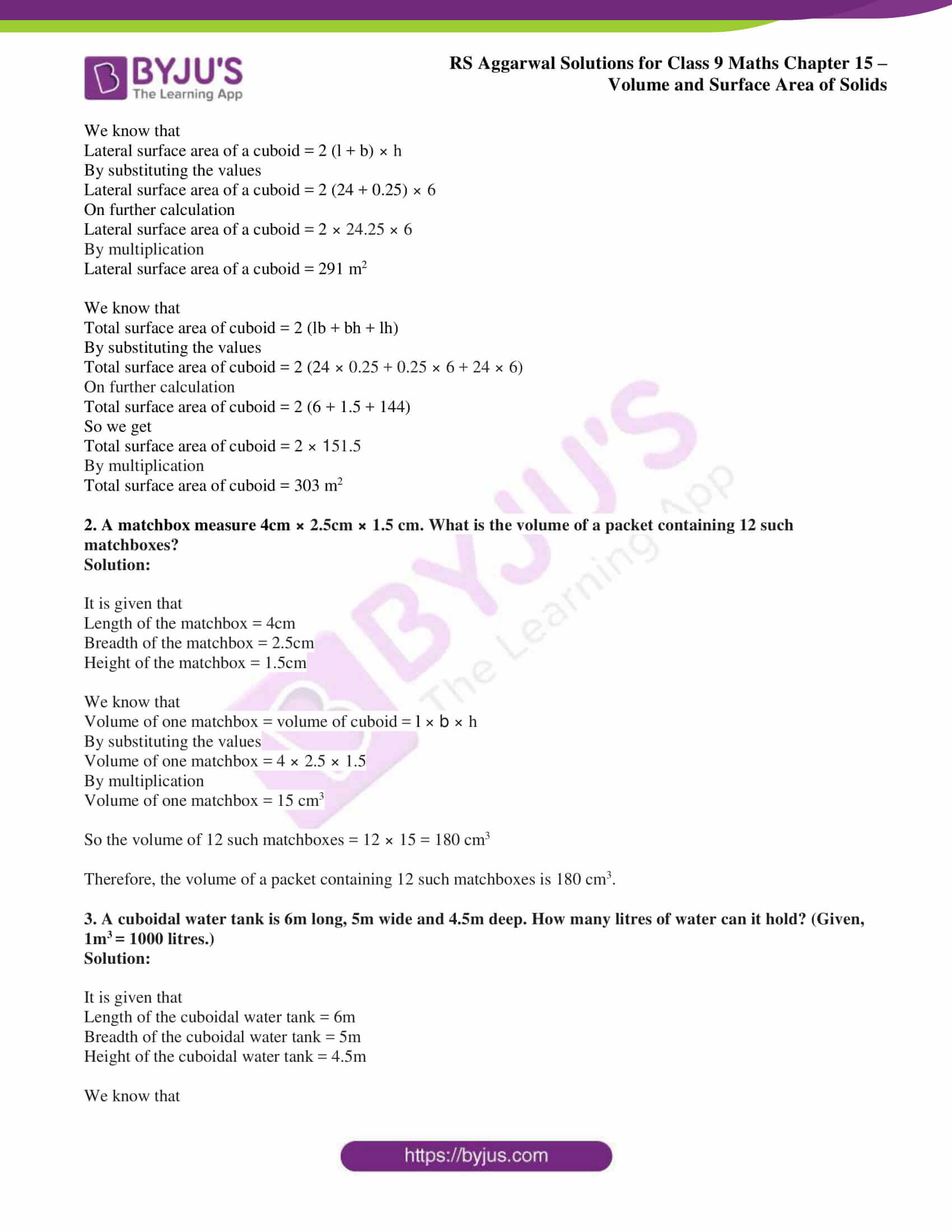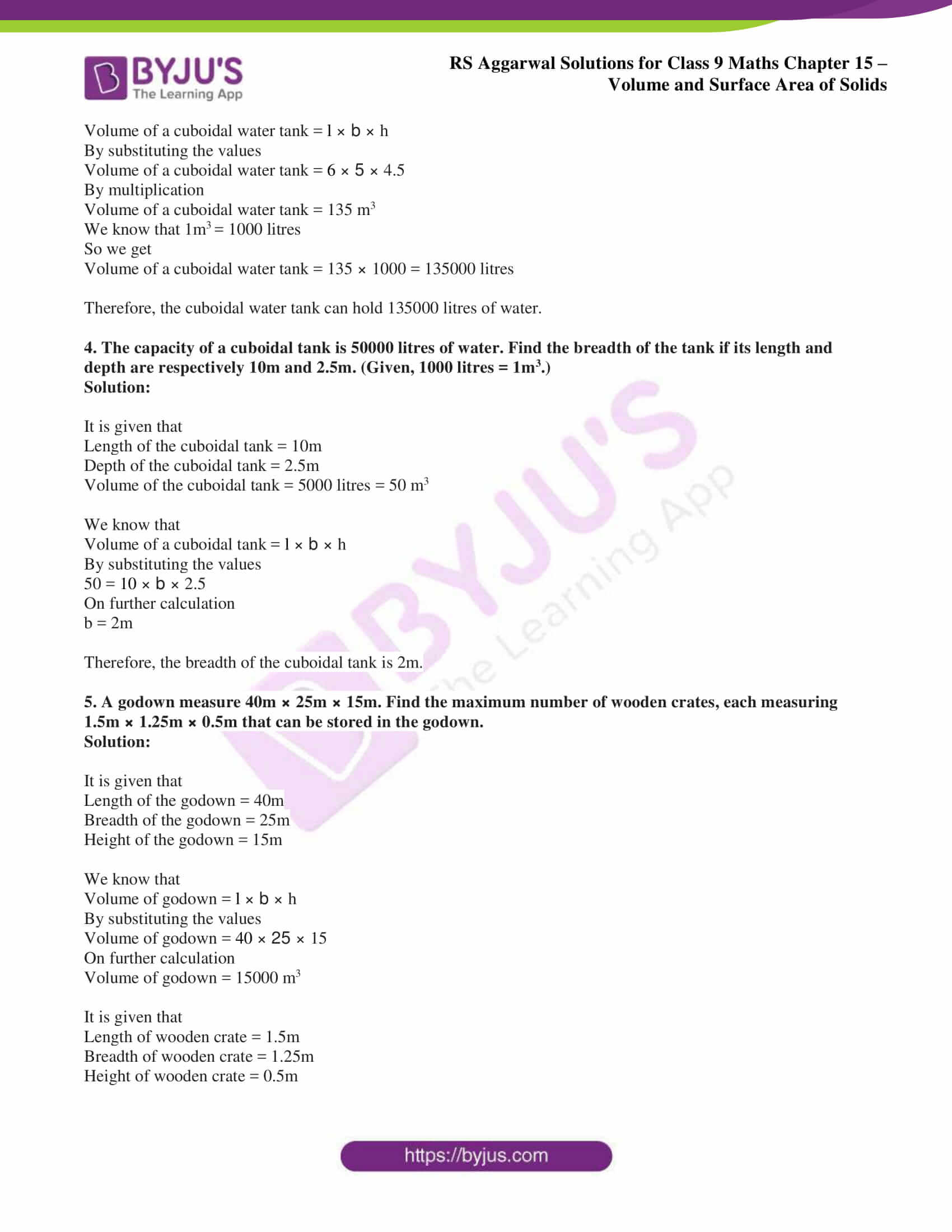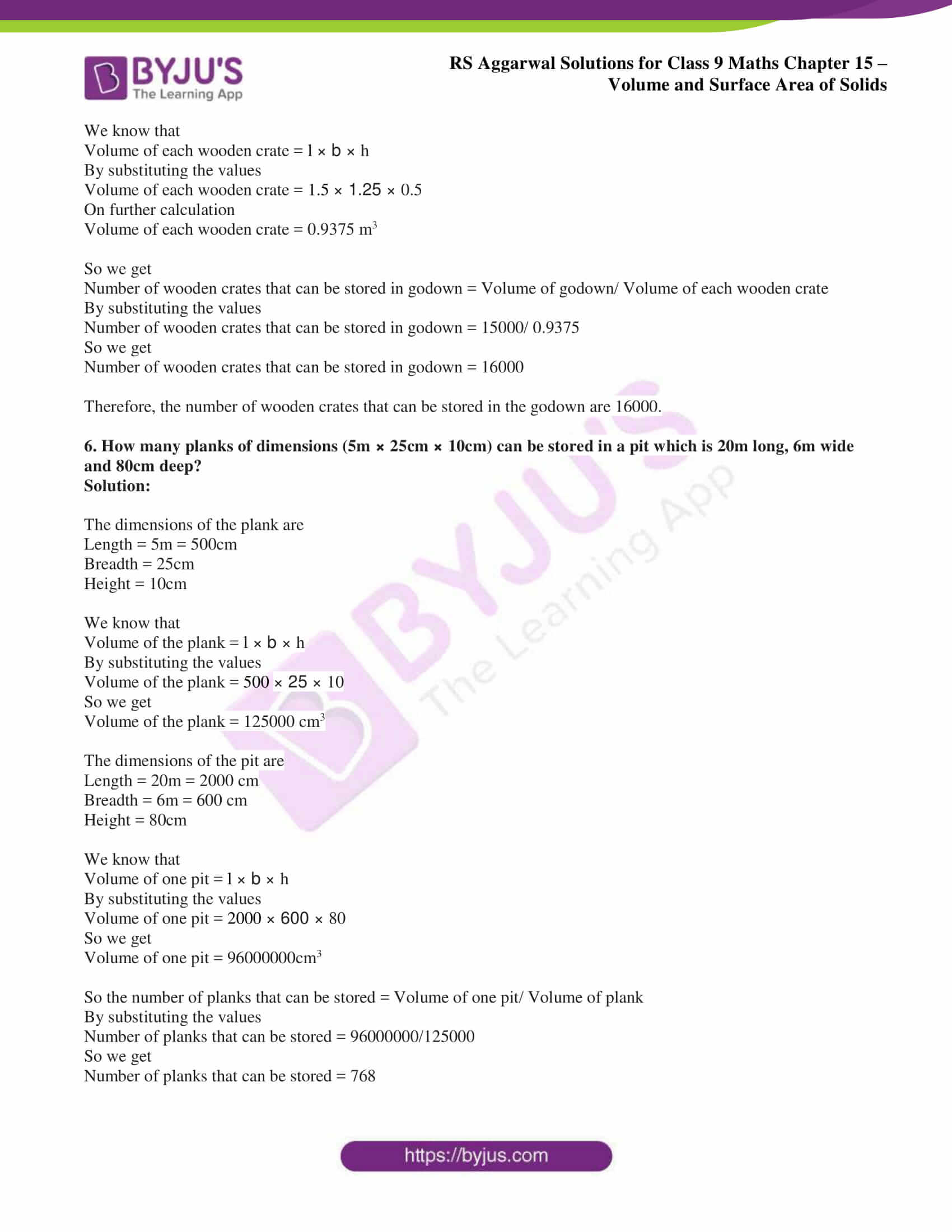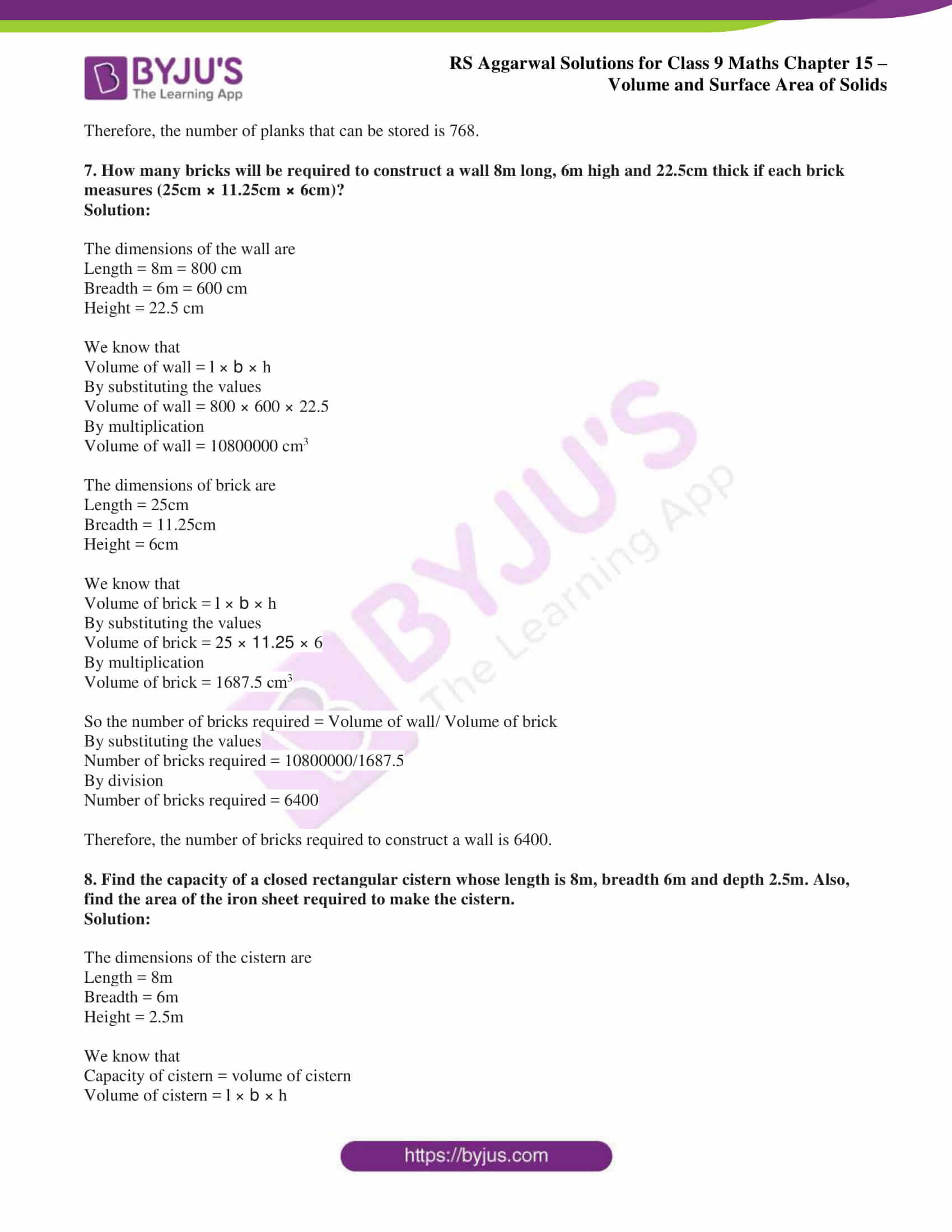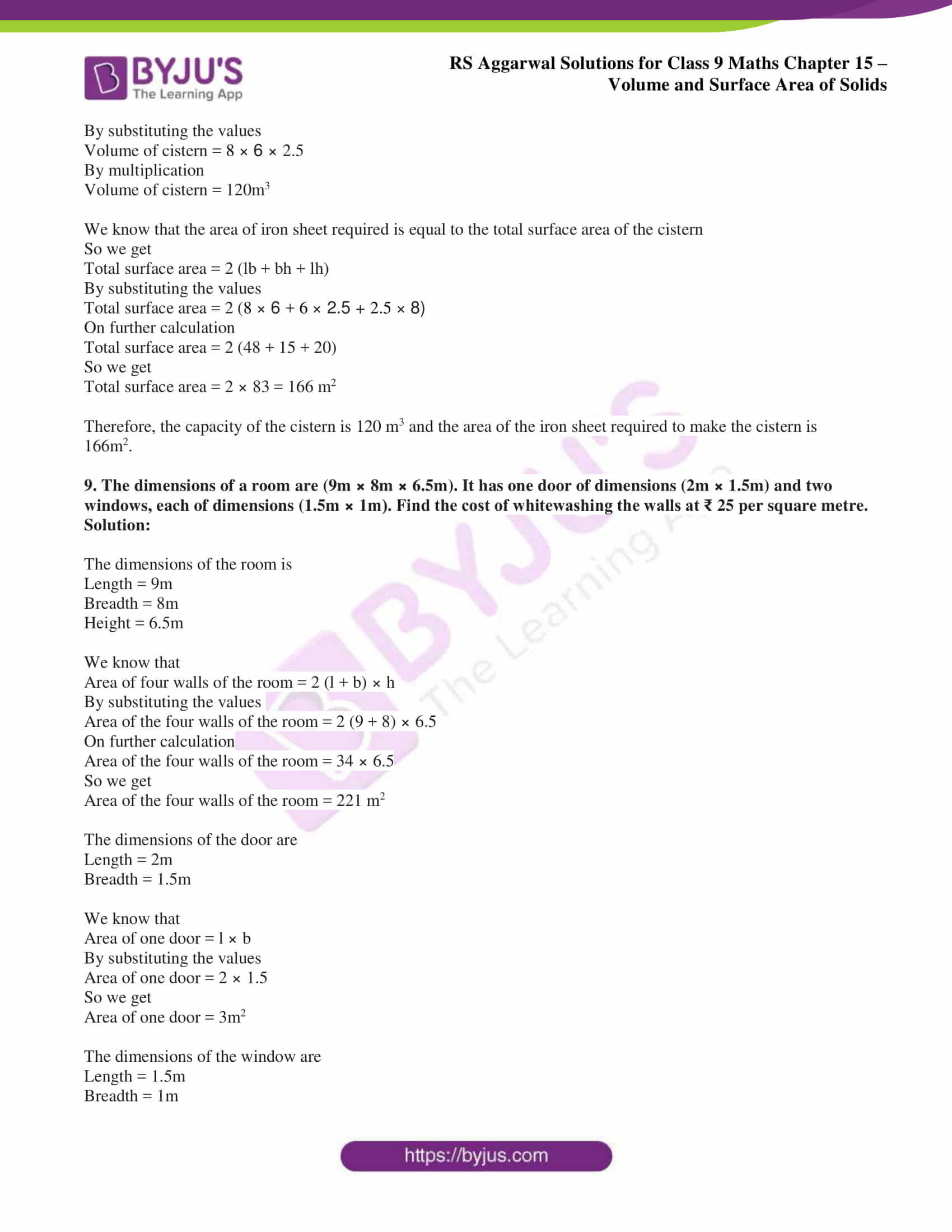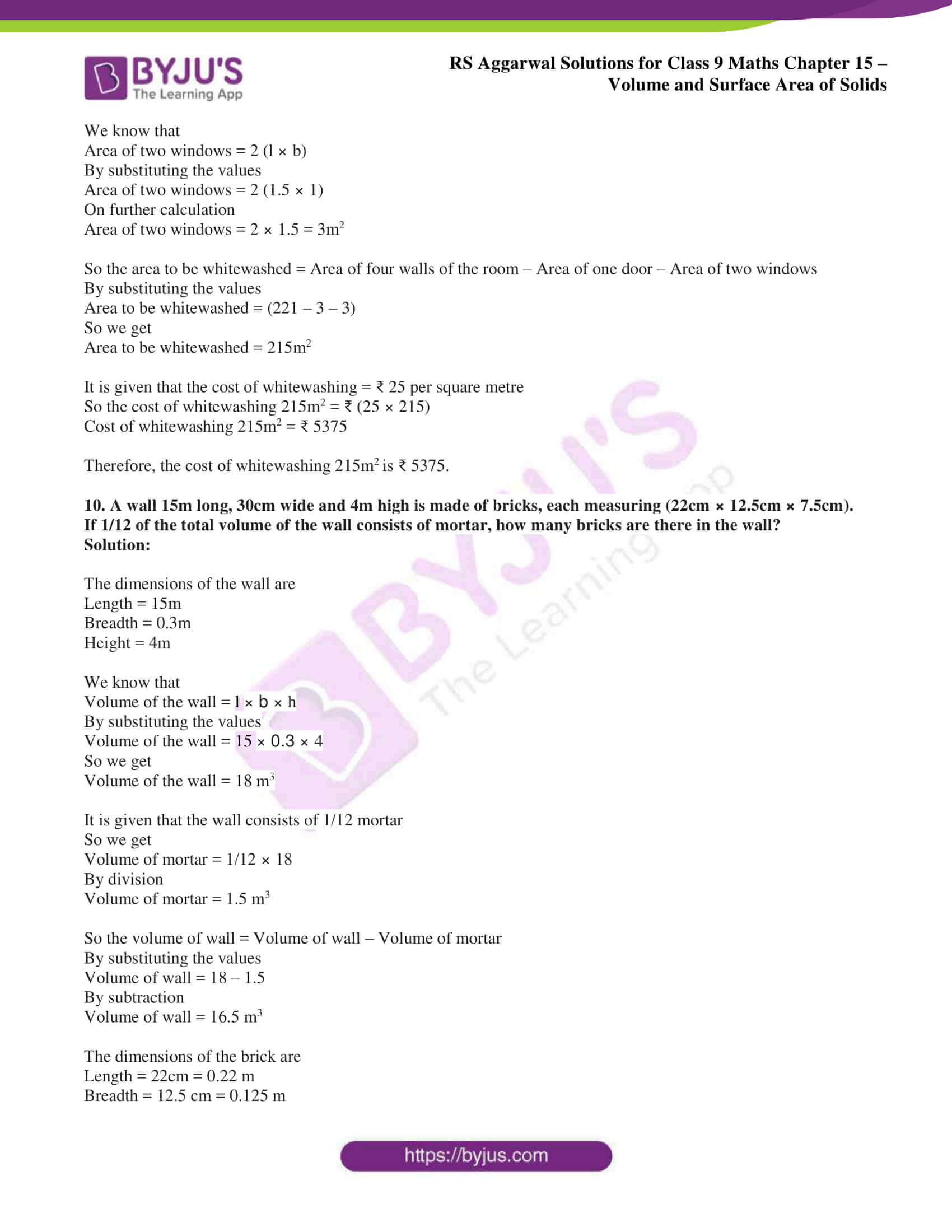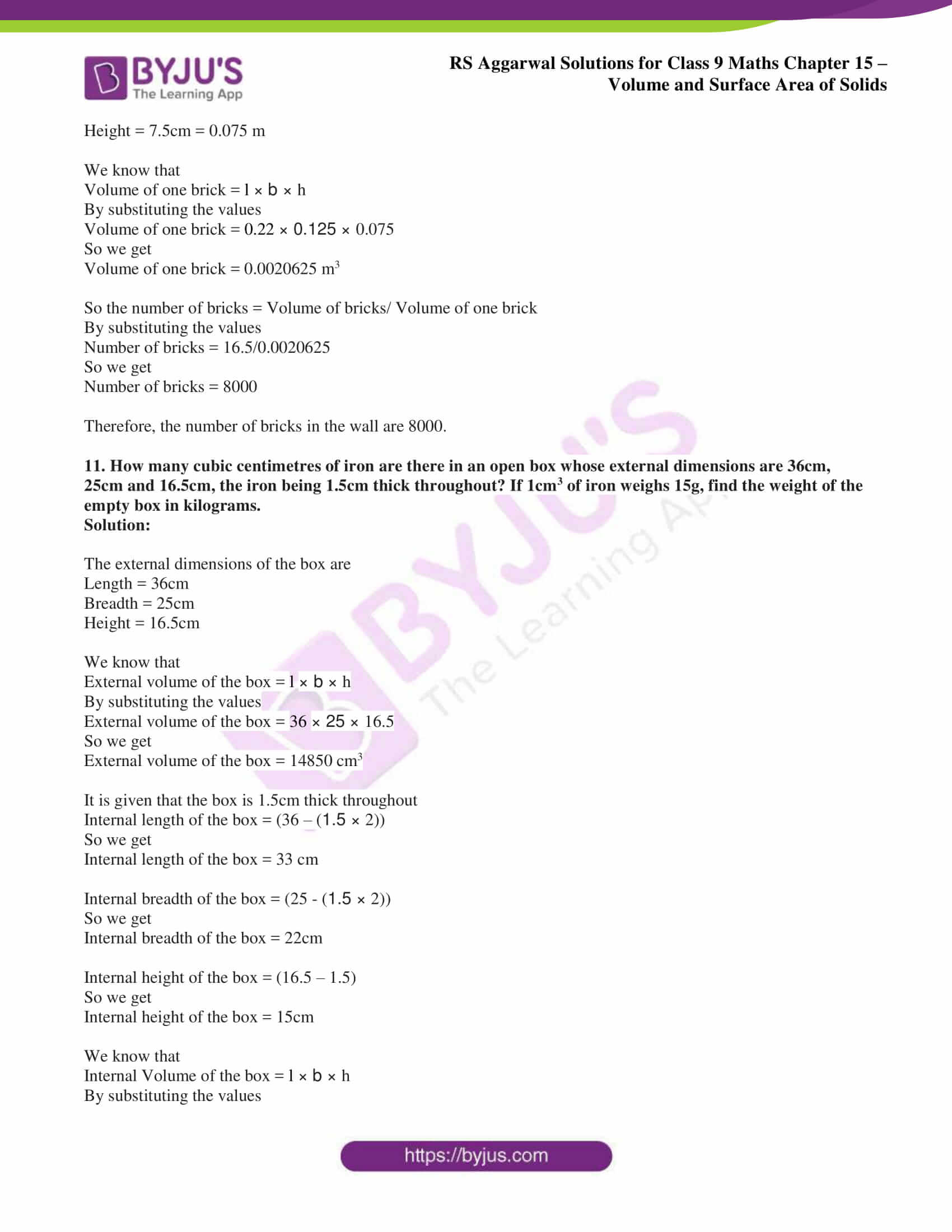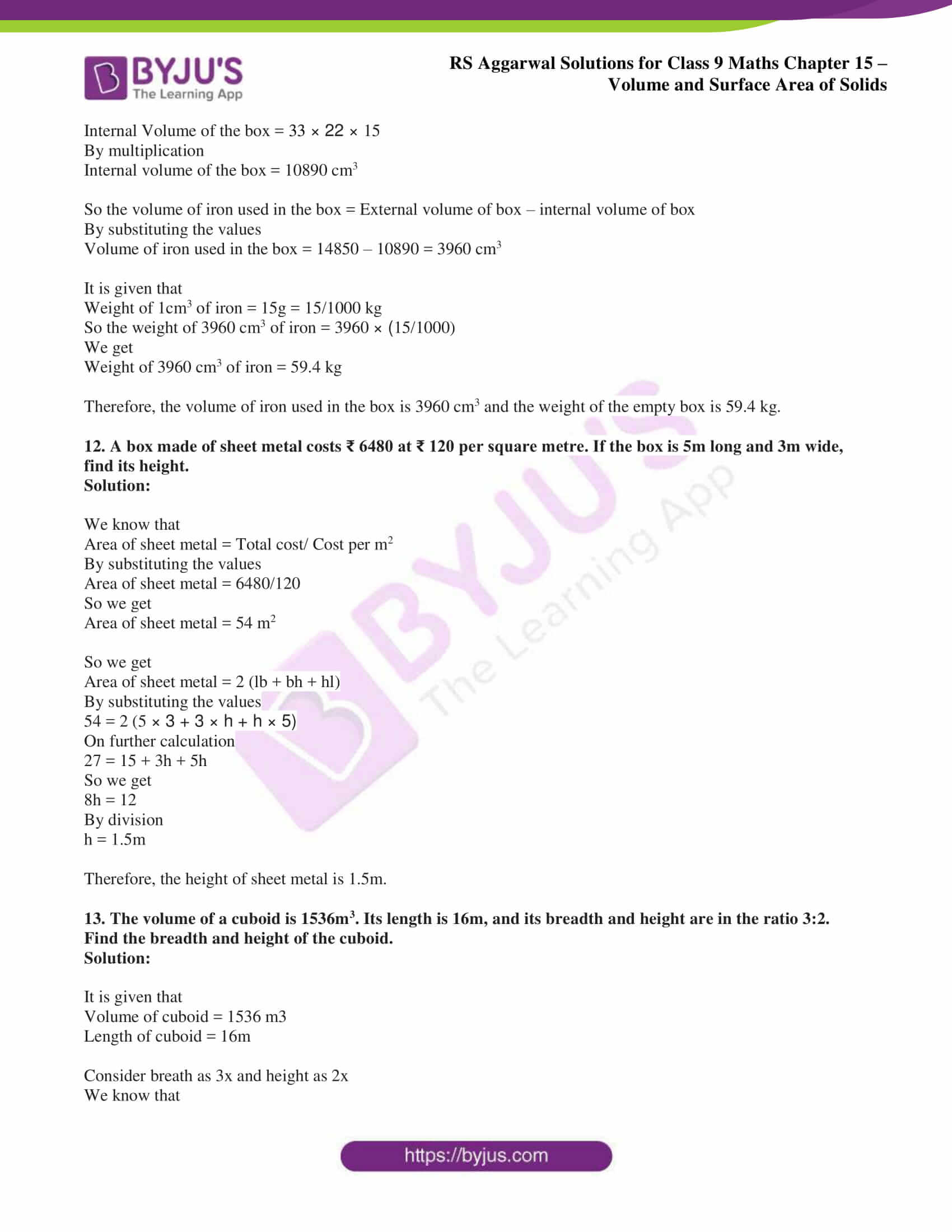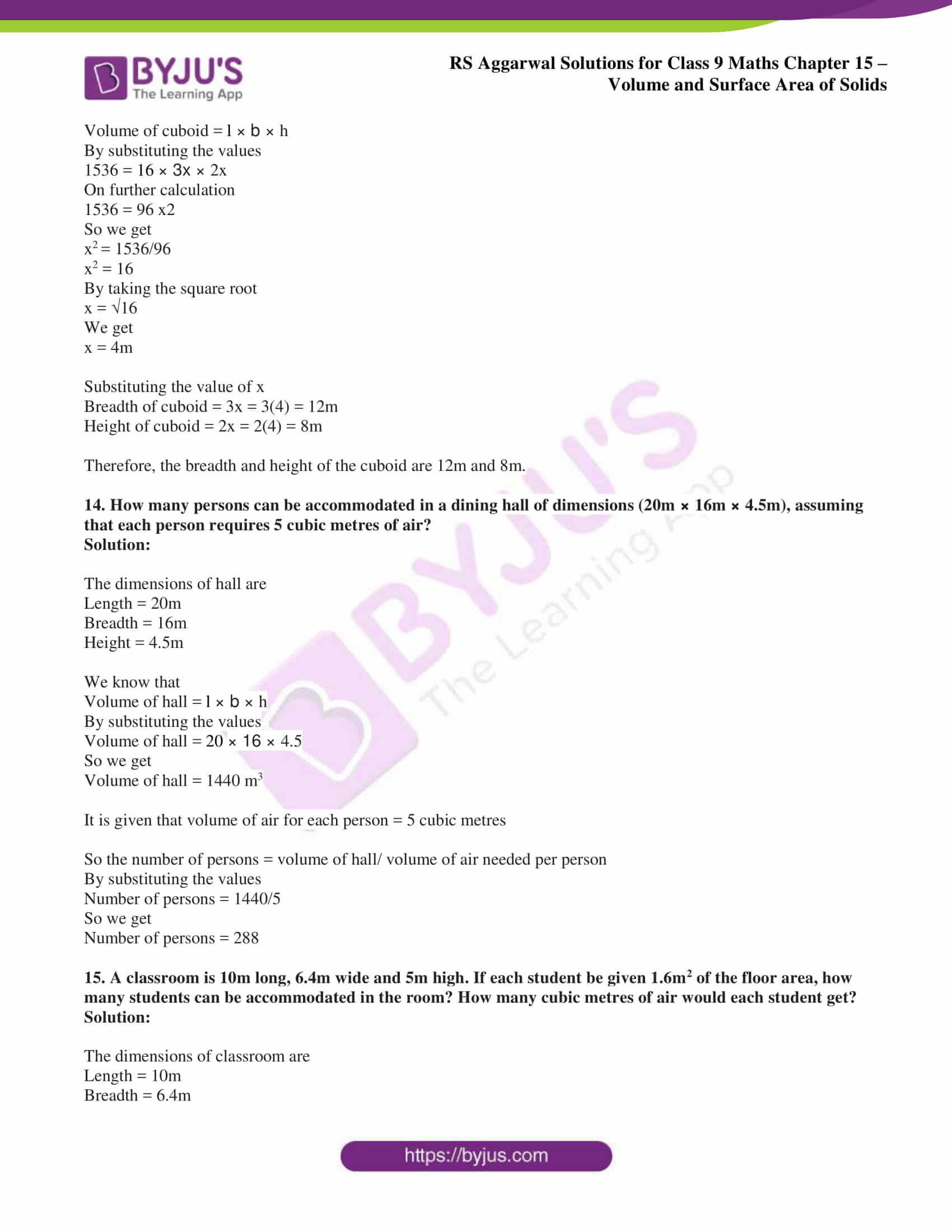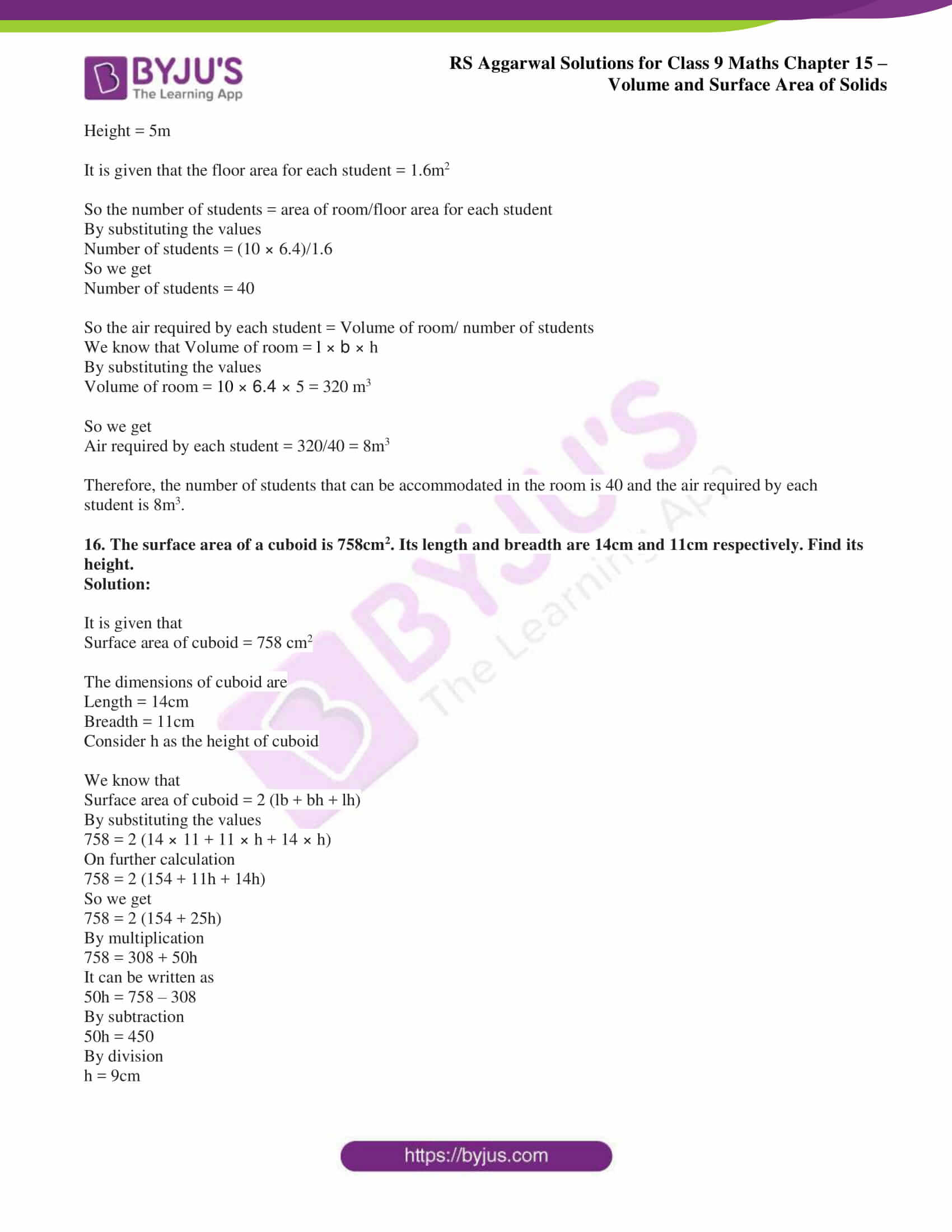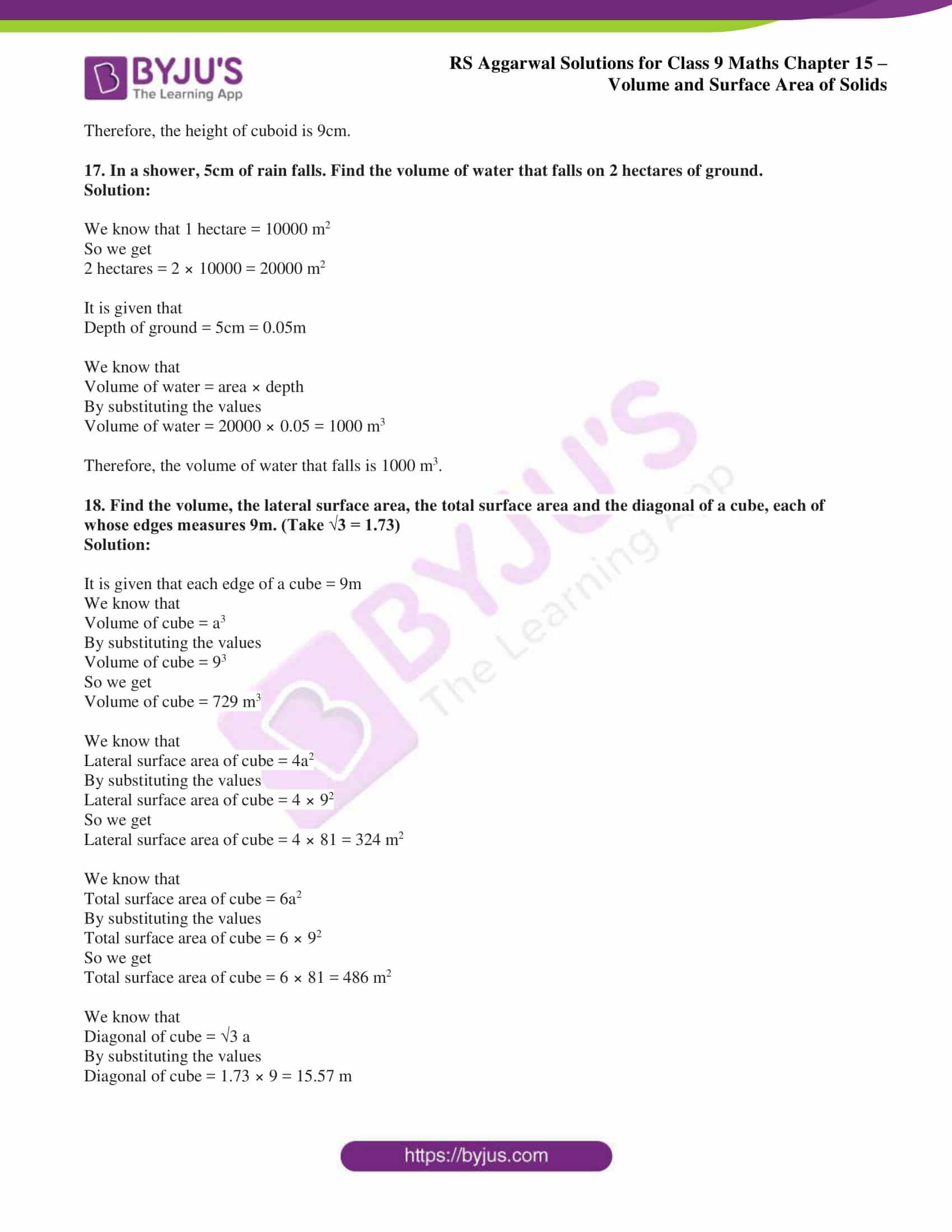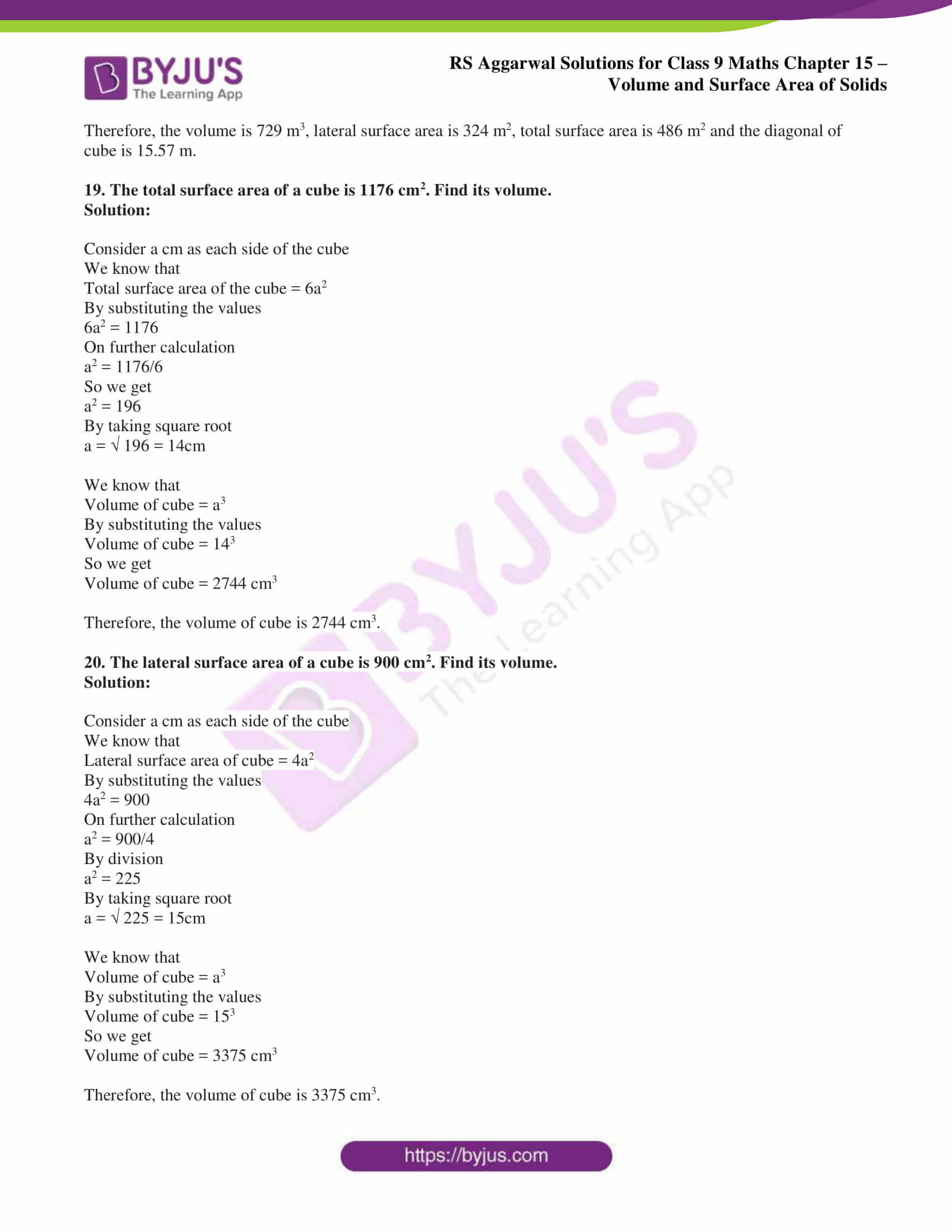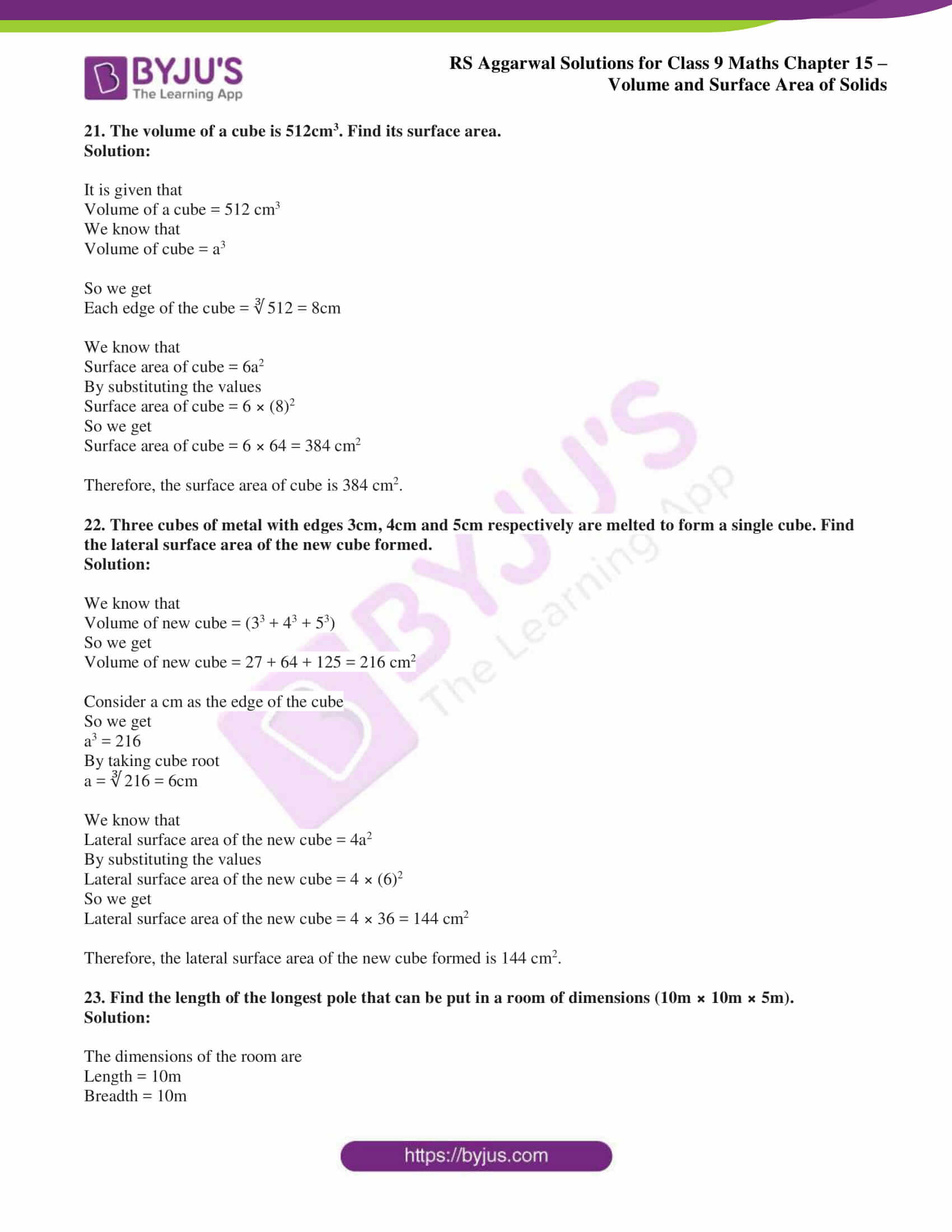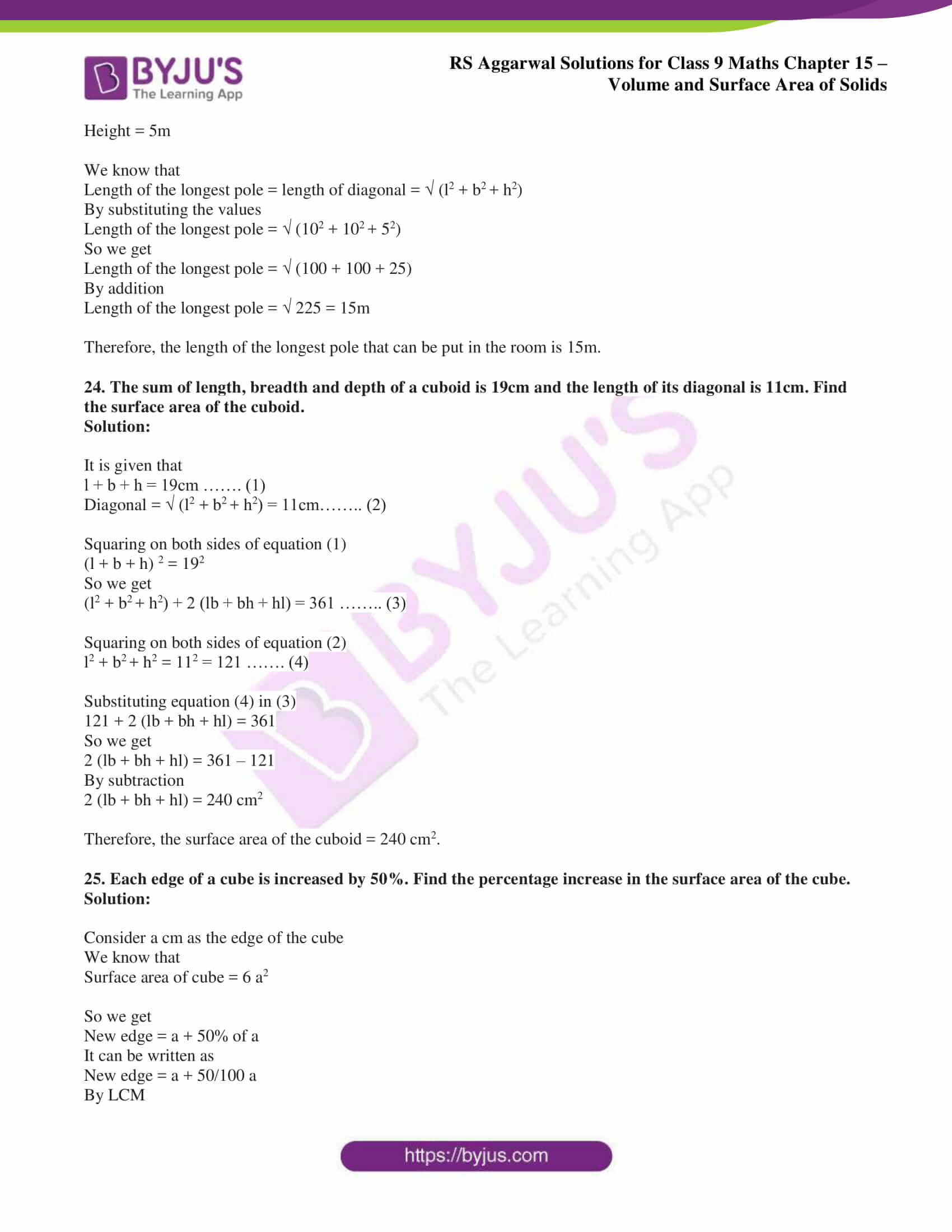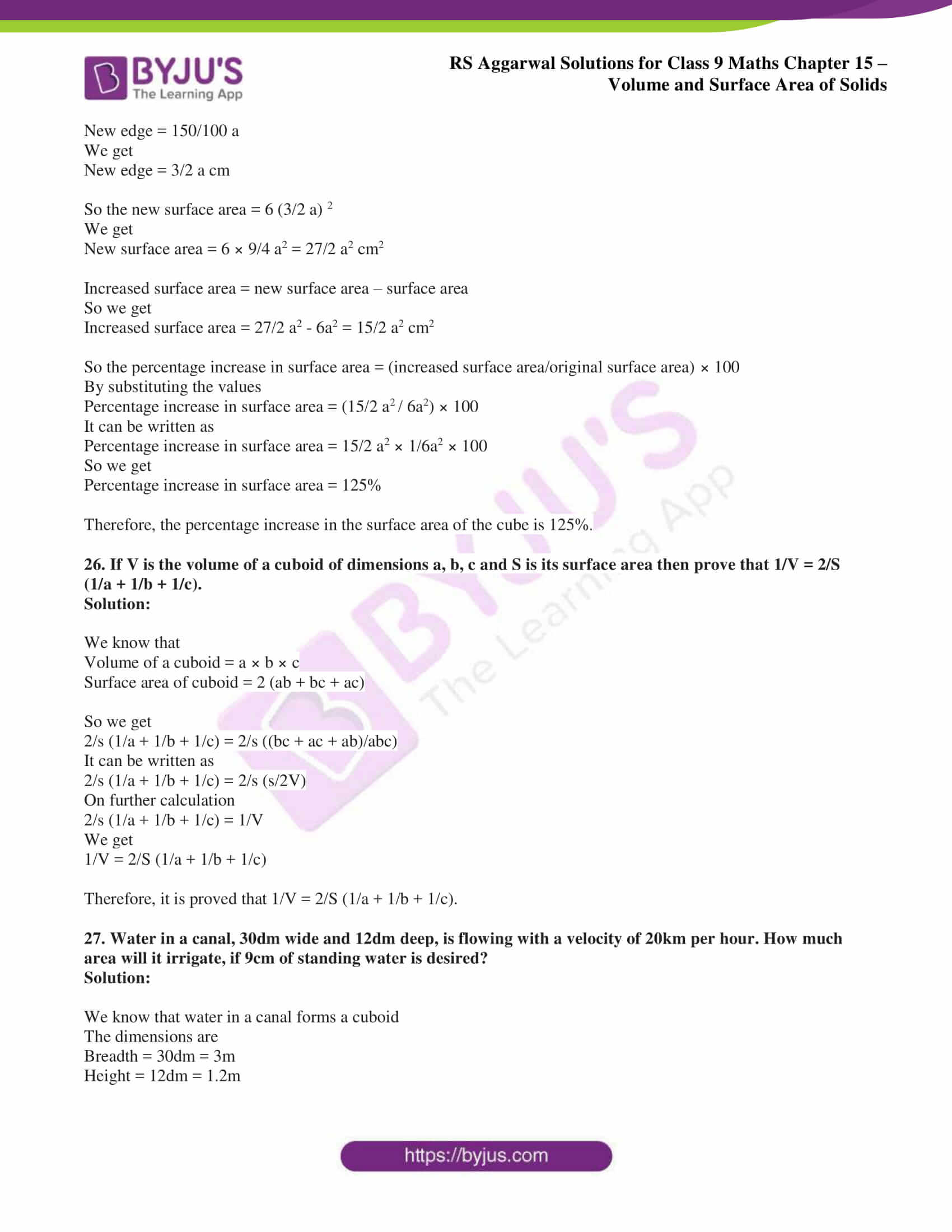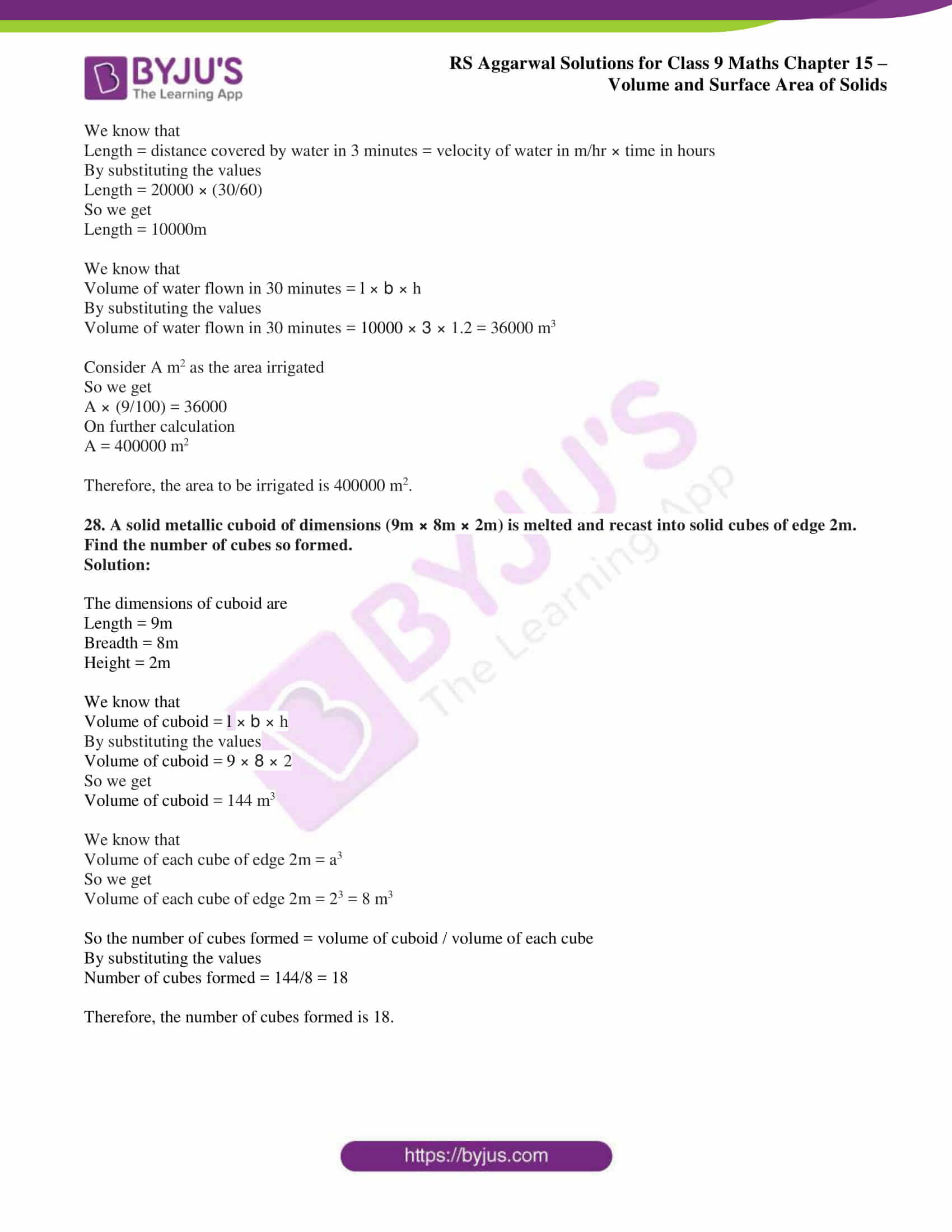## Access RS Aggarwal Solutions for Class 9 Chapter 15: Volumes and Surface Area of Solids Exercise 15A

1. Find the volume, the lateral surface area and the total surface area of the cuboid whose dimensions are:

(i) length = 12cm, breadth = 8cm and height = 4.5cm

(ii) length = 26m, breadth = 14m and height = 6.5m

(iii) length = 15m, breadth = 6m and height = 5dm

(iv) length = 24m, breadth = 25cm and height = 6m.

Solution:

(i) It is given that length = 12cm, breadth = 8cm and height = 4.5cm

We know that

Volume of cuboid = l × b × h

By substituting the values we get

Volume of cuboid = 12 × 8 × 4.5

By multiplication

Volume of cuboid = 432 cm3

We know that

Lateral surface area of a cuboid = 2 (l + b) × h

By substituting the values

Lateral surface area of a cuboid = 2 (12 + 8) × 4.5

On further calculation

Lateral surface area of a cuboid = 2 × 20 × 4.5

By multiplication

Lateral surface area of a cuboid = 180cm2

We know that

Total surface area of cuboid = 2 (lb + bh + lh)

By substituting the values

Total surface area of cuboid = 2 (12 × 8 + 8 × 4.5 + 12 × 4.5)

On further calculation

Total surface area of cuboid = 2 (96 + 36 + 54)

So we get

Total surface area of cuboid = 2 × 186

By multiplication

Total surface area of cuboid = 372 cm2

(ii) It is given that length = 26m, breadth = 14m and height = 6.5m

We know that

Volume of cuboid = l × b × h

By substituting the values we get

Volume of cuboid = 26 × 14 × 6.5

By multiplication

Volume of cuboid = 2366 m3

We know that

Lateral surface area of a cuboid = 2 (l + b) × h

By substituting the values

Lateral surface area of a cuboid = 2 (26 + 14) × 6.5

On further calculation

Lateral surface area of a cuboid = 2 × 40 × 6.5

By multiplication

Lateral surface area of a cuboid = 520cm2

We know that

Total surface area of cuboid = 2 (lb + bh + lh)

By substituting the values

Total surface area of cuboid = 2 (26 × 14 + 14 × 6.5 + 26 × 6.5)

On further calculation

Total surface area of cuboid = 2 (364 + 91 + 169)

So we get

Total surface area of cuboid = 2 × 624

By multiplication

Total surface area of cuboid = 1248 m2

(iii) It is given that length = 15m, breadth = 6m and height = 5dm = 0.5m

We know that

Volume of cuboid = l × b × h

By substituting the values we get

Volume of cuboid = 15 × 6 × 0.5

By multiplication

Volume of cuboid = 45 m3

We know that

Lateral surface area of a cuboid = 2 (l + b) × h

By substituting the values

Lateral surface area of a cuboid = 2 (15 + 6) × 0.5

On further calculation

Lateral surface area of a cuboid = 2 × 21 × 0.5

By multiplication

Lateral surface area of a cuboid = 21 m2

We know that

Total surface area of cuboid = 2 (lb + bh + lh)

By substituting the values

Total surface area of cuboid = 2 (15 × 6 + 6 × 0.5 + 15 × 0.5)

On further calculation

Total surface area of cuboid = 2 (90 + 3 + 7.5)

So we get

Total surface area of cuboid = 2 × 100.5

By multiplication

Total surface area of cuboid = 201 m2

(iv) It is given that length = 24m, breadth = 25cm = 0.25m and height = 6m

We know that

Volume of cuboid = l × b × h

By substituting the values we get

Volume of cuboid = 24 × 0.25 × 6

By multiplication

Volume of cuboid = 36 m3

We know that

Lateral surface area of a cuboid = 2 (l + b) × h

By substituting the values

Lateral surface area of a cuboid = 2 (24 + 0.25) × 6

On further calculation

Lateral surface area of a cuboid = 2 × 24.25 × 6

By multiplication

Lateral surface area of a cuboid = 291 m2

We know that

Total surface area of cuboid = 2 (lb + bh + lh)

By substituting the values

Total surface area of cuboid = 2 (24 × 0.25 + 0.25 × 6 + 24 × 6)

On further calculation

Total surface area of cuboid = 2 (6 + 1.5 + 144)

So we get

Total surface area of cuboid = 2 × 151.5

By multiplication

Total surface area of cuboid = 303 m2

2. A matchbox measure 4cm × 2.5cm × 1.5 cm. What is the volume of a packet containing 12 such matchboxes?

Solution:

It is given that

Length of the matchbox = 4cm

Breadth of the matchbox = 2.5cm

Height of the matchbox = 1.5cm

We know that

Volume of one matchbox = volume of cuboid = l × b × h

By substituting the values

Volume of one matchbox = 4 × 2.5 × 1.5

By multiplication

Volume of one matchbox = 15 cm3

So the volume of 12 such matchboxes = 12 × 15 = 180 cm3

Therefore, the volume of a packet containing 12 such matchboxes is 180 cm3.

3. A cuboidal water tank is 6m long, 5m wide and 4.5m deep. How many litres of water can it hold? (Given, 1m3 = 1000 litres.)

Solution:

It is given that

Length of the cuboidal water tank = 6m

Breadth of the cuboidal water tank = 5m

Height of the cuboidal water tank = 4.5m

We know that

Volume of a cuboidal water tank = l × b × h

By substituting the values

Volume of a cuboidal water tank = 6 × 5 × 4.5

By multiplication

Volume of a cuboidal water tank = 135 m3

We know that 1m3 = 1000 litres

So we get

Volume of a cuboidal water tank = 135 × 1000 = 135000 litres

Therefore, the cuboidal water tank can hold 135000 litres of water.

4. The capacity of a cuboidal tank is 50000 litres of water. Find the breadth of the tank if its length and depth are respectively 10m and 2.5m. (Given, 1000 litres = 1m3.)

Solution:

It is given that

Length of the cuboidal tank = 10m

Depth of the cuboidal tank = 2.5m

Volume of the cuboidal tank = 5000 litres = 50 m3

We know that

Volume of a cuboidal tank = l × b × h

By substituting the values

50 = 10 × b × 2.5

On further calculation

b = 2m

Therefore, the breadth of the cuboidal tank is 2m.

5. A godown measure 40m × 25m × 15m. Find the maximum number of wooden crates, each measuring 1.5m × 1.25m × 0.5m that can be stored in the godown.

Solution:

It is given that

Length of the godown = 40m

Breadth of the godown = 25m

Height of the godown = 15m

We know that

Volume of godown = l × b × h

By substituting the values

Volume of godown = 40 × 25 × 15

On further calculation

Volume of godown = 15000 m3

It is given that

Length of wooden crate = 1.5m

Breadth of wooden crate = 1.25m

Height of wooden crate = 0.5m

We know that

Volume of each wooden crate = l × b × h

By substituting the values

Volume of each wooden crate = 1.5 × 1.25 × 0.5

On further calculation

Volume of each wooden crate = 0.9375 m3

So we get

Number of wooden crates that can be stored in godown = Volume of godown/ Volume of each wooden crate

By substituting the values

Number of wooden crates that can be stored in godown = 15000/ 0.9375

So we get

Number of wooden crates that can be stored in godown = 16000

Therefore, the number of wooden crates that can be stored in the godown are 16000.

6. How many planks of dimensions (5m × 25cm × 10cm) can be stored in a pit which is 20m long, 6m wide and 80cm deep?

Solution:

The dimensions of the plank are

Length = 5m = 500cm

Height = 10cm

We know that

Volume of the plank = l × b × h

By substituting the values

Volume of the plank = 500 × 25 × 10

So we get

Volume of the plank = 125000 cm3

The dimensions of the pit are

Length = 20m = 2000 cm

Breadth = 6m = 600 cm

Height = 80cm

We know that

Volume of one pit = l × b × h

By substituting the values

Volume of one pit = 2000 × 600 × 80

So we get

Volume of one pit = 96000000cm3

So the number of planks that can be stored = Volume of one pit/ Volume of plank

By substituting the values

Number of planks that can be stored = 96000000/125000

So we get

Number of planks that can be stored = 768

Therefore, the number of planks that can be stored is 768.

7. How many bricks will be required to construct a wall 8m long, 6m high and 22.5cm thick if each brick measures (25cm × 11.25cm × 6cm)?

Solution:

The dimensions of the wall are

Length = 8m = 800 cm

Breadth = 6m = 600 cm

Height = 22.5 cm

We know that

Volume of wall = l × b × h

By substituting the values

Volume of wall = 800 × 600 × 22.5

By multiplication

Volume of wall = 10800000 cm3

The dimensions of brick are

Length = 25cm

Height = 6cm

We know that

Volume of brick = l × b × h

By substituting the values

Volume of brick = 25 × 11.25 × 6

By multiplication

Volume of brick = 1687.5 cm3

So the number of bricks required = Volume of wall/ Volume of brick

By substituting the values

Number of bricks required = 10800000/1687.5

By division

Number of bricks required = 6400

Therefore, the number of bricks required to construct a wall is 6400.

8. Find the capacity of a closed rectangular cistern whose length is 8m, breadth 6m and depth 2.5m. Also, find the area of the iron sheet required to make the cistern.

Solution:

The dimensions of the cistern are

Length = 8m

Height = 2.5m

We know that

Capacity of cistern = volume of cistern

Volume of cistern = l × b × h

By substituting the values

Volume of cistern = 8 × 6 × 2.5

By multiplication

Volume of cistern = 120m3

We know that the area of iron sheet required is equal to the total surface area of the cistern

So we get

Total surface area = 2 (lb + bh + lh)

By substituting the values

Total surface area = 2 (8 × 6 + 6 × 2.5 + 2.5 × 8)

On further calculation

Total surface area = 2 (48 + 15 + 20)

So we get

Total surface area = 2 × 83 = 166 m2

Therefore, the capacity of the cistern is 120 m3 and the area of the iron sheet required to make the cistern is 166m2.

9. The dimensions of a room are (9m × 8m × 6.5m). It has one door of dimensions (2m × 1.5m) and two windows, each of dimensions (1.5m × 1m). Find the cost of whitewashing the walls at ₹ 25 per square metre.

Solution:

The dimensions of the room is

Length = 9m

Height = 6.5m

We know that

Area of four walls of the room = 2 (l + b) × h

By substituting the values

Area of the four walls of the room = 2 (9 + 8) × 6.5

On further calculation

Area of the four walls of the room = 34 × 6.5

So we get

Area of the four walls of the room = 221 m2

The dimensions of the door are

Length = 2m

We know that

Area of one door = l × b

By substituting the values

Area of one door = 2 × 1.5

So we get

Area of one door = 3m2

The dimensions of the window are

Length = 1.5m

We know that

Area of two windows = 2 (l × b)

By substituting the values

Area of two windows = 2 (1.5 × 1)

On further calculation

Area of two windows = 2 × 1.5 = 3m2

So the area to be whitewashed = Area of four walls of the room – Area of one door – Area of two windows

By substituting the values

Area to be whitewashed = (221 – 3 – 3)

So we get

Area to be whitewashed = 215m2

It is given that the cost of whitewashing = ₹ 25 per square metre

So the cost of whitewashing 215m2 = ₹ (25 × 215)

Cost of whitewashing 215m2 = ₹ 5375

Therefore, the cost of whitewashing 215m2 is ₹ 5375.

10. A wall 15m long, 30cm wide and 4m high is made of bricks, each measuring (22cm × 12.5cm × 7.5cm). If 1/12 of the total volume of the wall consists of mortar, how many bricks are there in the wall?

Solution:

The dimensions of the wall are

Length = 15m

Height = 4m

We know that

Volume of the wall = l × b × h

By substituting the values

Volume of the wall = 15 × 0.3 × 4

So we get

Volume of the wall = 18 m3

It is given that the wall consists of 1/12 mortar

So we get

Volume of mortar = 1/12 × 18

By division

Volume of mortar = 1.5 m3

So the volume of wall = Volume of wall – Volume of mortar

By substituting the values

Volume of wall = 18 – 1.5

By subtraction

Volume of wall = 16.5 m3

The dimensions of the brick are

Length = 22cm = 0.22 m

Breadth = 12.5 cm = 0.125 m

Height = 7.5cm = 0.075 m

We know that

Volume of one brick = l × b × h

By substituting the values

Volume of one brick = 0.22 × 0.125 × 0.075

So we get

Volume of one brick = 0.0020625 m3

So the number of bricks = Volume of bricks/ Volume of one brick

By substituting the values

Number of bricks = 16.5/0.0020625

So we get

Number of bricks = 8000

Therefore, the number of bricks in the wall are 8000.

11. How many cubic centimetres of iron are there in an open box whose external dimensions are 36cm, 25cm and 16.5cm, the iron being 1.5cm thick throughout? If 1cm3 of iron weighs 15g, find the weight of the empty box in kilograms.

Solution:

The external dimensions of the box are

Length = 36cm

Height = 16.5cm

We know that

External volume of the box = l × b × h

By substituting the values

External volume of the box = 36 × 25 × 16.5

So we get

External volume of the box = 14850 cm3

It is given that the box is 1.5cm thick throughout

Internal length of the box = (36 – (1.5 × 2))

So we get

Internal length of the box = 33 cm

Internal breadth of the box = (25 – (1.5 × 2))

So we get

Internal breadth of the box = 22cm

Internal height of the box = (16.5 – 1.5)

So we get

Internal height of the box = 15cm

We know that

Internal Volume of the box = l × b × h

By substituting the values

Internal Volume of the box = 33 × 22 × 15

By multiplication

Internal volume of the box = 10890 cm3

So the volume of iron used in the box = External volume of box – internal volume of box

By substituting the values

Volume of iron used in the box = 14850 – 10890 = 3960 cm3

It is given that

Weight of 1cm3 of iron = 15g = 15/1000 kg

So the weight of 3960 cm3 of iron = 3960 × (15/1000)

We get

Weight of 3960 cm3 of iron = 59.4 kg

Therefore, the volume of iron used in the box is 3960 cm3 and the weight of the empty box is 59.4 kg.

12. A box made of sheet metal costs ₹ 6480 at ₹ 120 per square metre. If the box is 5m long and 3m wide, find its height.

Solution:

We know that

Area of sheet metal = Total cost/ Cost per m2

By substituting the values

Area of sheet metal = 6480/120

So we get

Area of sheet metal = 54 m2

So we get

Area of sheet metal = 2 (lb + bh + hl)

By substituting the values

54 = 2 (5 × 3 + 3 × h + h × 5)

On further calculation

27 = 15 + 3h + 5h

So we get

8h = 12

By division

h = 1.5m

Therefore, the height of sheet metal is 1.5m.

13. The volume of a cuboid is 1536m3. Its length is 16m, and its breadth and height are in the ratio 3:2. Find the breadth and height of the cuboid.

Solution:

It is given that

Volume of cuboid = 1536 m3

Length of cuboid = 16m

Consider breath as 3x and height as 2x

We know that

Volume of cuboid = l × b × h

By substituting the values

1536 = 16 × 3x × 2x

On further calculation

1536 = 96 x2

So we get

x2 = 1536/96

x2 = 16

By taking the square root

x = √16

We get

x = 4m

Substituting the value of x

Breadth of cuboid = 3x = 3(4) = 12m

Height of cuboid = 2x = 2(4) = 8m

Therefore, the breadth and height of the cuboid are 12m and 8m.

14. How many persons can be accommodated in a dining hall of dimensions (20m × 16m × 4.5m), assuming that each person requires 5 cubic metres of air?

Solution:

The dimensions of hall are

Length = 20m

Height = 4.5m

We know that

Volume of hall = l × b × h

By substituting the values

Volume of hall = 20 × 16 × 4.5

So we get

Volume of hall = 1440 m3

It is given that volume of air for each person = 5 cubic metres

So the number of persons = volume of hall/ volume of air needed per person

By substituting the values

Number of persons = 1440/5

So we get

Number of persons = 288

15. A classroom is 10m long, 6.4m wide and 5m high. If each student be given 1.6m2 of the floor area, how many students can be accommodated in the room? How many cubic metres of air would each student get?

Solution:

The dimensions of classroom are

Length = 10m

Height = 5m

It is given that the floor area for each student = 1.6m2

So the number of students = area of room/floor area for each student

By substituting the values

Number of students = (10 × 6.4)/1.6

So we get

Number of students = 40

So the air required by each student = Volume of room/ number of students

We know that Volume of room = l × b × h

By substituting the values

Volume of room = 10 × 6.4 × 5 = 320 m3

So we get

Air required by each student = 320/40 = 8m3

Therefore, the number of students that can be accommodated in the room is 40 and the air required by each student is 8m3.

16. The surface area of a cuboid is 758cm2. Its length and breadth are 14cm and 11cm respectively. Find its height.

Solution:

It is given that

Surface area of cuboid = 758 cm2

The dimensions of cuboid are

Length = 14cm

Consider h as the height of cuboid

We know that

Surface area of cuboid = 2 (lb + bh + lh)

By substituting the values

758 = 2 (14 × 11 + 11 × h + 14 × h)

On further calculation

758 = 2 (154 + 11h + 14h)

So we get

758 = 2 (154 + 25h)

By multiplication

758 = 308 + 50h

It can be written as

50h = 758 – 308

By subtraction

50h = 450

By division

h = 9cm

Therefore, the height of cuboid is 9cm.

17. In a shower, 5cm of rain falls. Find the volume of water that falls on 2 hectares of ground.

Solution:

We know that 1 hectare = 10000 m2

So we get

2 hectares = 2 × 10000 = 20000 m2

It is given that

Depth of ground = 5cm = 0.05m

We know that

Volume of water = area × depth

By substituting the values

Volume of water = 20000 × 0.05 = 1000 m3

Therefore, the volume of water that falls is 1000 m3.

18. Find the volume, the lateral surface area, the total surface area and the diagonal of a cube, each of whose edges measures 9m. (Take √3 = 1.73)

Solution:

It is given that each edge of a cube = 9m

We know that

Volume of cube = a3

By substituting the values

Volume of cube = 93

So we get

Volume of cube = 729 m3

We know that

Lateral surface area of cube = 4a2

By substituting the values

Lateral surface area of cube = 4 × 92

So we get

Lateral surface area of cube = 4 × 81 = 324 m2

We know that

Total surface area of cube = 6a2

By substituting the values

Total surface area of cube = 6 × 92

So we get

Total surface area of cube = 6 × 81 = 486 m2

We know that

Diagonal of cube = √3 a

By substituting the values

Diagonal of cube = 1.73 × 9 = 15.57 m

Therefore, the volume is 729 m3, lateral surface area is 324 m2, total surface area is 486 m2 and the diagonal of cube is 15.57 m.

19. The total surface area of a cube is 1176 cm2. Find its volume.

Solution:

Consider a cm as each side of the cube

We know that

Total surface area of the cube = 6a2

By substituting the values

6a2 = 1176

On further calculation

a2 = 1176/6

So we get

a2 = 196

By taking square root

a = √ 196 = 14cm

We know that

Volume of cube = a3

By substituting the values

Volume of cube = 143

So we get

Volume of cube = 2744 cm3

Therefore, the volume of cube is 2744 cm3.

20. The lateral surface area of a cube is 900 cm2. Find its volume.

Solution:

Consider a cm as each side of the cube

We know that

Lateral surface area of cube = 4a2

By substituting the values

4a2 = 900

On further calculation

a2 = 900/4

By division

a2 = 225

By taking square root

a = √ 225 = 15cm

We know that

Volume of cube = a3

By substituting the values

Volume of cube = 153

So we get

Volume of cube = 3375 cm3

Therefore, the volume of cube is 3375 cm3.

21. The volume of a cube is 512cm3. Find its surface area.

Solution:

It is given that

Volume of a cube = 512 cm3

We know that

Volume of cube = a3

So we get

Each edge of the cube = ∛ 512 = 8cm

We know that

Surface area of cube = 6a2

By substituting the values

Surface area of cube = 6 × (8)2

So we get

Surface area of cube = 6 × 64 = 384 cm2

Therefore, the surface area of cube is 384 cm2.

22. Three cubes of metal with edges 3cm, 4cm and 5cm respectively are melted to form a single cube. Find the lateral surface area of the new cube formed.

Solution:

We know that

Volume of new cube = (33 + 43 + 53)

So we get

Volume of new cube = 27 + 64 + 125 = 216 cm2

Consider a cm as the edge of the cube

So we get

a3 = 216

By taking cube root

a = ∛ 216 = 6cm

We know that

Lateral surface area of the new cube = 4a2

By substituting the values

Lateral surface area of the new cube = 4 × (6)2

So we get

Lateral surface area of the new cube = 4 × 36 = 144 cm2

Therefore, the lateral surface area of the new cube formed is 144 cm2.

23. Find the length of the longest pole that can be put in a room of dimensions (10m × 10m × 5m).

Solution:

The dimensions of the room are

Length = 10m

Height = 5m

We know that

Length of the longest pole = length of diagonal = √ (l2 + b2 + h2)

By substituting the values

Length of the longest pole = √ (102 + 102 + 52)

So we get

Length of the longest pole = √ (100 + 100 + 25)

Length of the longest pole = √ 225 = 15m

Therefore, the length of the longest pole that can be put in the room is 15m.

24. The sum of length, breadth and depth of a cuboid is 19cm and the length of its diagonal is 11cm. Find the surface area of the cuboid.

Solution:

It is given that

l + b + h = 19cm ……. (1)

Diagonal = √ (l2 + b2 + h2) = 11cm…….. (2)

Squaring on both sides of equation (1)

(l + b + h) 2 = 192

So we get

(l2 + b2 + h2) + 2 (lb + bh + hl) = 361 …….. (3)

Squaring on both sides of equation (2)

l2 + b2 + h2 = 112 = 121 ……. (4)

Substituting equation (4) in (3)

121 + 2 (lb + bh + hl) = 361

So we get

2 (lb + bh + hl) = 361 – 121

By subtraction

2 (lb + bh + hl) = 240 cm2

Therefore, the surface area of the cuboid = 240 cm2.

25. Each edge of a cube is increased by 50%. Find the percentage increase in the surface area of the cube.

Solution:

Consider a cm as the edge of the cube

We know that

Surface area of cube = 6 a2

So we get

New edge = a + 50% of a

It can be written as

New edge = a + 50/100 a

By LCM

New edge = 150/100 a

We get

New edge = 3/2 a cm

So the new surface area = 6 (3/2 a) 2

We get

New surface area = 6 × 9/4 a2 = 27/2 a2 cm2

Increased surface area = new surface area – surface area

So we get

Increased surface area = 27/2 a2 – 6a2 = 15/2 a2 cm2

So the percentage increase in surface area = (increased surface area/original surface area) × 100

By substituting the values

Percentage increase in surface area = (15/2 a2 / 6a2) × 100

It can be written as

Percentage increase in surface area = 15/2 a2 × 1/6a2 × 100

So we get

Percentage increase in surface area = 125%

Therefore, the percentage increase in the surface area of the cube is 125%.

26. If V is the volume of a cuboid of dimensions a, b, c and S is its surface area then prove that 1/V = 2/S (1/a + 1/b + 1/c).

Solution:

We know that

Volume of a cuboid = a × b × c

Surface area of cuboid = 2 (ab + bc + ac)

So we get

2/s (1/a + 1/b + 1/c) = 2/s ((bc + ac + ab)/abc)

It can be written as

2/s (1/a + 1/b + 1/c) = 2/s (s/2V)

On further calculation

2/s (1/a + 1/b + 1/c) = 1/V

We get

1/V = 2/S (1/a + 1/b + 1/c)

Therefore, it is proved that 1/V = 2/S (1/a + 1/b + 1/c).

27. Water in a canal, 30dm wide and 12dm deep, is flowing with a velocity of 20km per hour. How much area will it irrigate, if 9cm of standing water is desired?

Solution:

We know that water in a canal forms a cuboid

The dimensions are

Height = 12dm = 1.2m

We know that

Length = distance covered by water in 3 minutes = velocity of water in m/hr × time in hours

By substituting the values

Length = 20000 × (30/60)

So we get

Length = 10000m

We know that

Volume of water flown in 30 minutes = l × b × h

By substituting the values

Volume of water flown in 30 minutes = 10000 × 3 × 1.2 = 36000 m3

Consider A m2 as the area irrigated

So we get

A × (9/100) = 36000

On further calculation

A = 400000 m2

Therefore, the area to be irrigated is 400000 m2.

28. A solid metallic cuboid of dimensions (9m × 8m × 2m) is melted and recast into solid cubes of edge 2m. Find the number of cubes so formed.

Solution:

The dimensions of cuboid are

Length = 9m

Height = 2m

We know that

Volume of cuboid = l × b × h

By substituting the values

Volume of cuboid = 9 × 8 × 2

So we get

Volume of cuboid = 144 m3

We know that

Volume of each cube of edge 2m = a3

So we get

Volume of each cube of edge 2m = 23 = 8 m3

So the number of cubes formed = volume of cuboid / volume of each cube

By substituting the values

Number of cubes formed = 144/8 = 18

Therefore, the number of cubes formed is 18.

### Access other exercise solutions of Class 9 Maths Chapter 15: Volume and Surface Area of Solids

Exercise 15B Solutions 33 Questions

Exercise 15C Solutions 21 Questions

Exercise 15D Solutions 28 Questions

### RS Aggarwal Solutions Class 9 Maths Chapter 15 – Volume and Surface Area of Solids Exercise 15A

RS Aggarwal Solutions Class 9 Maths Chapter 15 Volume and Surface Area of Solids Exercise 15A explains the formulas and methods which are used to find volume and surface area of cube and cuboid.

### Key features of RS Aggarwal Solutions for Class 9 Maths Chapter 15: Volume and Surface Area of Solids Exercise 15A

• The solutions contain list of important formulas which can be used in finding the surface area and volume.
• Shortcuts and tips are mentioned on ways to help students solve problems within the specified duration.
• The main aim is to provide the students with effortless solutions which help in solving problems.
• The step by step solutions are designed by keeping in mind the latest syllabus of CBSE board.